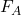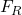Question

A 150kg motorcycle starts from rest and accelerates at a constant rate along with a distance of 350m. The applied force is 250N and the coefficient of kinetic friction is 0.03. What is the net force applied to the motorcycle?

1.ngochoa

205N

Explanation:

The net force (F) is the difference between the applied force() and the kinetic frictional force(). i.e

F =—————–(i)

Note that;= μmg

Where;

μ = coefficient of kinetic friction

m = mass of the body

g = acceleration due to gravity = 10m/s²

Equation (i) then becomes;

F =– μmg        ——————-(ii)

Given from question;

m = mass of motorcycle = 150kg

μ = 0.03= 250N

Substitute these values into equation (ii) as follows;

F = 250 – (0.03 x 150 x 10)

F = 250 – (45)

F = 205N

Therefore, the net force applied to the motorcycle is 205N